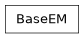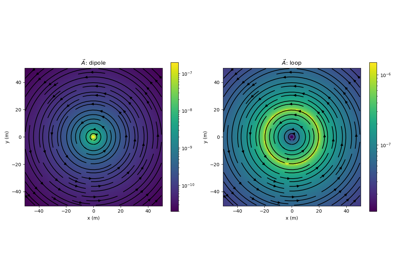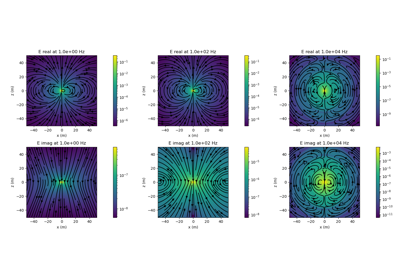# geoana.em.BaseEM#class geoana.em.BaseEM(sigma=1.0, mu=1.25663706212e-06, epsilon=8.8541878128e-12, **kwargs)#

Base electromagnetics class.

The base EM class stores the physical properties that are relevant to all problems which solve Maxwell’s equations. The base EM class assumes that all physical properties (conductivity, magnetic permeability and dielectric permittivity) are homogeneously distributed within a wholespace and are non-dispersive. These properties are overwritten in child classes as necessary.

Parameters
sigmafloat, optional

Electrical conductivity in S/m. Default is $$\sigma$$ = 1 S/m

mufloat, optional

Magnetic permeability in H/m. Default is $$\mu_0 = 4\pi \times 10^{-7}$$ H/m

epsilonfloat, optional

Dielectric permittivity F/m. Default is $$\epsilon_0 = 8.85 \times 10^{-12}$$ F/m

Attributes

 epsilon Dielectric permittivity in F/m mu Magnetic permeability in H/m sigma Electrical conductivity in S/m

## Galleries and Tutorials using geoana.em.BaseEM#Magnetostatic Vector Potentials: Dipole and Loop Sources

Magnetostatic Vector Potentials: Dipole and Loop SourcesTotal magnetic fields: Dipole and Pole sources

Total magnetic fields: Dipole and Pole sourcesElectric Dipole in a Whole Space: Frequency Domain

Electric Dipole in a Whole Space: Frequency Domain